Courses

# Similarity between Triangles and Polygons Mathematics Notes | EduRev

## Mathematics : Similarity between Triangles and Polygons Mathematics Notes | EduRev

The document Similarity between Triangles and Polygons Mathematics Notes | EduRev is a part of the Mathematics Course Additional Topics for IIT JAM Mathematics.
All you need of Mathematics at this link: Mathematics

Polygons
Any two polygons are similar if their corresponding angles are congruent and the measures of their corresponding sides are proportional: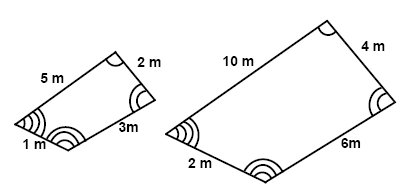In the figure above the ratio or the scale factor of the quadrilateral to the left versus the quadrilateral to the right is ½. We could also turn it around and say that the scale factor for the quadrilateral to the right to the quadrilateral to the left is 2.

Triangles
If the measures of the corresponding sides of two triangles are proportional then the triangles are similar. Likewise if the measures of two sides in one triangle are proportional to the corresponding sides in another triangle and the including angles are congruent then the triangles are similar.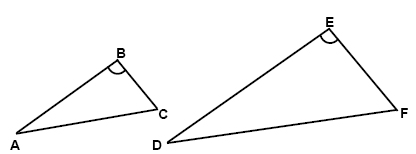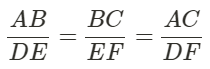If a line is drawn in a triangle so that it is parallel to one of the sides and it intersects the other two sides then the segments are of proportional lengths: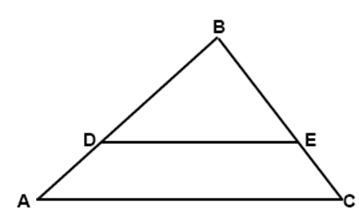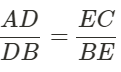Parts of two triangles can be proportional; if two triangles are known to be similar then the perimeters are proportional to the measures of corresponding sides.
Continuing, if two triangles are known to be similar then the measures of the corresponding altitudes are proportional to the corresponding sides.
Lastly, if two triangles are known to be similar then the measures of the corresponding angle bisectors or the corresponding medians are proportional to the measures of the corresponding sides.
The bisector of an angle in a triangle separates the opposite side into two segments that have the same ratio as the other two sides: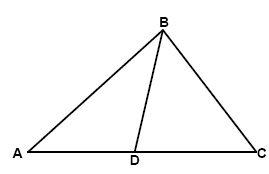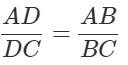Offer running on EduRev: Apply code STAYHOME200 to get INR 200 off on our premium plan EduRev Infinity!

40 docs

,

,

,

,

,

,

,

,

,

,

,

,

,

,

,

,

,

,

,

,

,

;